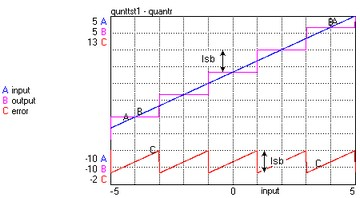﻿ 20-sim webhelp > Library > Signal > Discrete > Quantize-Round

Quantize-Round

Signal\Discrete

Use

Domains: Discrete, Continuous. Size: 1-D. Allowed in: Block Diagrams.

Description

This model can be used to represent quantization of an input signal. It rounds the input as shown in the graph below. The quantization interval is specified by the least significant bit (lsb). The maximum output error is half a least significant bit.Note

Compare this model with the model Quantisize-Truncate which represents quantization by truncation. Truncation results in a maximum error of a least significant bit.

Inputs

Description

input

output

Parameters

lsb

Least significant bit.